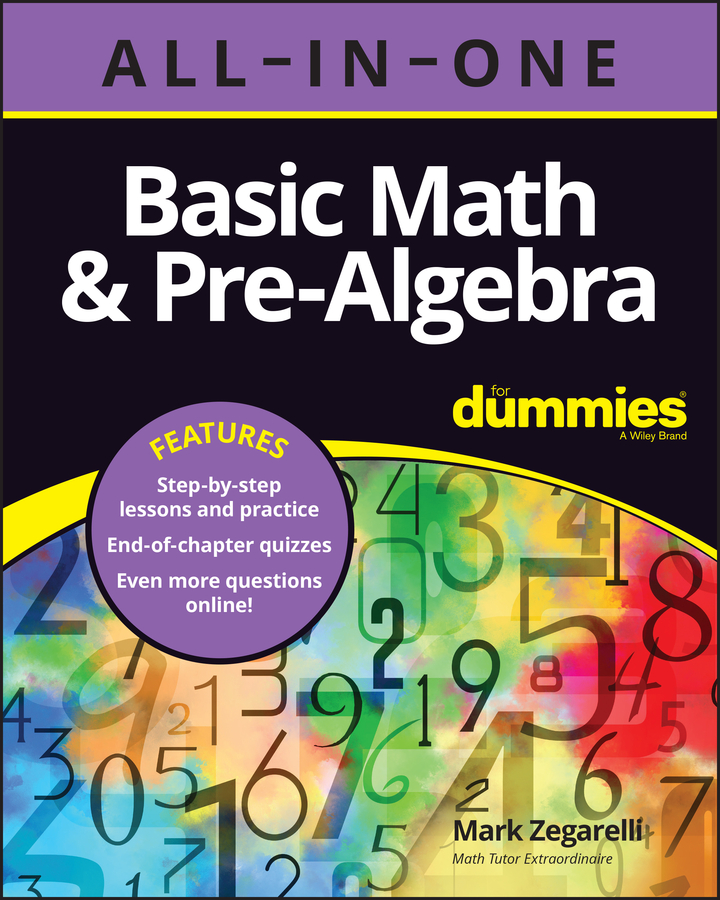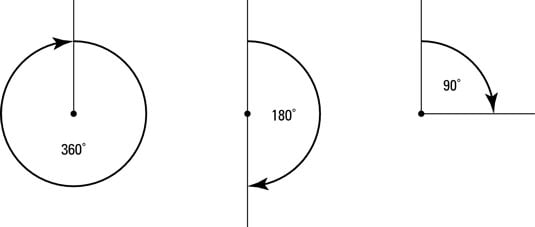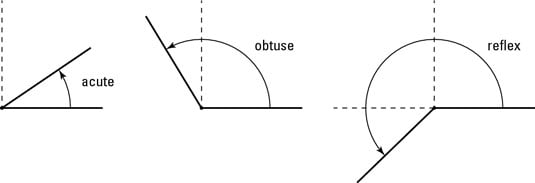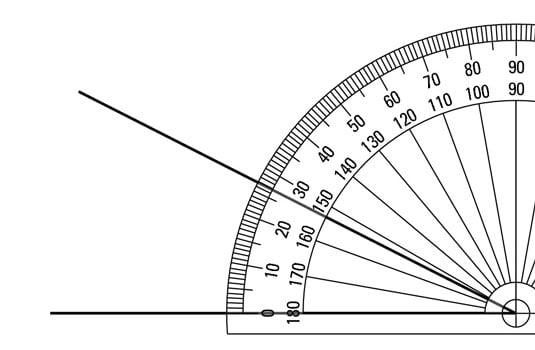##### Basic Math & Pre-Algebra All-in-One For Dummies (+ Chapter Quizzes Online)Angle is another word for corner. For example, you may hear of footballers scoring from tight angles, meaning they’ve turned the ball round a sharp corner. The word ‘angle’ also shows up in other words, such as ‘triangle’ – which simply means ‘three corners’.

Angles are measured in degrees. For example, if a car spins 360 degrees, it spins all the way round, while the latitude of London is 53 degrees north of the equator. Confusingly, angle degrees are completely different from temperature degrees – the context usually makes clear which type of degrees you need to work with (except possibly when talking about pointy icicles!). Both types of degree are denoted by a little circle above and after the number, for example: 90º.

Angles are interesting for many reasons, but one of the key points is that their properties don’t really depend on how big the lines leading to the corner are. For example, the angle on a bookend is the same whether it’s a bookend for tiny books or huge books – in either case, the angle is 90 degrees.

## Defining angles

When someone says an angle is a certain number of degrees, they’re trying to tell you how sharp the corner is. A small angle means the corner is very sharp, while an angle of 180 degrees isn’t much of an angle at all but instead is a straight line.

One degree is defined as ‘one three-hundred-and-sixtieth of a circle’, which isn’t tremendously helpful (but then again, you may not find the technical definitions of metres and kilograms helpful either).

### Special angles

You need to know about the following special angles:
• 360 degrees is a complete circle. If you turn around 360 degrees, you get back to where you started.

• 180 degrees is half a circle. If you turn 180 degrees, you end up facing backwards.

• 90 degrees is a quarter-circle, or a right-angle. If you turn 90 degrees, you end up facing to the left or the right of where you started.### Other types of angle

You need to know the following angle-related words:
• An acute angle is an angle smaller than a right-angle – so less than 90 degrees. Think of ‘acute little puppy’ to remind yourself that it’s a little angle.

• A reflex angle is an angle bigger than 180 degrees. You know when your doctor hits the outside of your knee with a hammer to test your reflexes? Think of that to remember what a reflex angle is: the outside of your knee is always more than 180 degrees.

• An obtuse angle is in between – so more than 90 degrees but less than 180.## Measuring angles

You measure angles with a protractor, one of those semi-circular things you had in your pencil case at school.

Here’s how you measure an angle with a protractor:

1. Put the cross-hair in the middle of your protractor over the corner where you want to measure the angle.

2. Turn the protractor so that one of the lines going into the angle is on the ‘zero’ line across the bottom of the protractor, to the left of the cross-hair.

3. Follow the other line to the edge of the protractor and read the number off the scale – that’s the angle you’re looking for.Protractors are fiddly because they have two different scales running along the same edge and you can easily mix up which one is which. Always use the outside scale – so make sure the zero you use is the zero on the outside track of the protractor.

After you measure an angle, look at the angle again and ask whether it makes sense. If you have an angle that’s obviously more than 90 degrees (an obtuse angle) but your protractor says the angle measures 15 degrees, you know something has gone wrong.